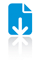# A General Purpose Algorithm for Counting Simple Cycles and Simple Paths of Any Length

ContentAbstract

We describe a general purpose algorithm for counting simple cycles and simple paths of any length ℓ on a (weighted di)graph on N vertices and M edges, achieving an asymptotic running time of O(N+M+(ℓω+ℓΔ)|Sℓ|). In this expression, |Sℓ| is the number of (weakly) connected induced subgraphs of G on at most ℓ vertices, Δ is the maximum degree of any vertex and ω is the exponent of matrix multiplication. We compare the algorithm complexity both theoretically and experimentally with most of the existing algorithms for the same task. These comparisons show that the algorithm described here is the best general purpose algorithm for the class of graphs where (ℓω−1Δ−1+1)|Sℓ|≤|Cycleℓ|, with |Cycleℓ| the total number of simple cycles of length at most ℓ, including backtracks and self-loops. On Erdős–Rényi random graphs, we find empirically that this happens when the edge probability is larger than circa 4 / N. In addition, we show that some real-world networks also belong to this class. Finally, the algorithm permits the enumeration of simple cycles and simple paths on networks where vertices are labeled from an alphabet on n letters with an asymptotic running time of O(N+M+(nℓℓω+ℓΔ)|Sℓ|). A Matlab implementation of the algorithm proposed here is available for download.Top
Authors
• Giscard, Pierre-Louis
• Kriege, Nils M.
• Wilson, Richard C.Top
Shortfacts
 Category Journal Paper Divisions Journal or Publication Title Algorithmica ISSN 0178-4617 Publisher Springer Page Range pp. 2716-2737 Number 7 Volume 81 Date 15 February 2019 Export BibTeX ASCII Citation DIDL Dublin Core EP3 XML EP3 XML with files embedded EndNote Eprints Application Profile HTML Citation JSON METS MODS Multiline CSV OAI-ORE Resource Map (Atom Format) OAI-ORE Resource Map (RDF Format) OpenURL ContextObject RDF+N-Triples RDF+N3 RDF+XML Refer Reference Manager Simple MetadataTop